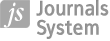ORIGINAL PAPER
Computational Approach to Solving a Layered Behaviour Differential Equation with Large Delay Using Quadrature Scheme
,

,

More details
Hide details
1
Department of Mathematics, University College of Science, Osmania University, Hyderabad, India

2
Department of Mathematics, Malla Reddy Engineering College, Hyderabad

Online publication date: 2022-12-03

Publication date: 2022-12-01

International Journal of Applied Mechanics and Engineering 2022;27(4):117–137

KEYWORDS
ABSTRACT
This paper deals with the computational approach to solving the singularly perturbed differential equation with a large delay in the differentiated term using the two-point Gaussian quadrature. If the delay is bigger than the perturbed parameter, the layer behaviour of the solution is destroyed, and the solution becomes oscillatory. With the help of a special type mesh, a numerical scheme consisting of a fitting parameter is developed to minimize the error and to control the layer structure in the solution. The scheme is studied for convergence. Compared with other methods in the literature, the maximum defects in the approach are tabularized to validate the competency of the numerical approach. In the suggested technique, we additionally focused on the effect of a large delay on the layer structure or oscillatory behaviour of the solutions using a special form of mesh with and without a fitting parameter. The effect of the fitting parameter is demonstrated in graphs to show its impact on the layer of the solution.

REFERENCES (22)
1.
Bellman R. and Cooke K.L. (1963): Differential-Difference Equations.– New York: Academic Press.

2.
Bestehornand M. and Grigoriev E.V. (2004): Formation and propagation of localized states in extended systems.– Ann. Phys., vol.13, No.8, pp.423-431.

3.
Derstine M.W., Gibbs H.M., Hopf F.A. and Kaplan D.L. (1982): Bifurcation gap in a hybrid optical system.– Phys. Rev. A., vol.26, pp.3720-3722.

4.
Kuang Y. (1993): Delay Differential Equations with Applications in Population Dynamics.– New York: Academic Press.

5.
Mackey M.C. and Glass L. (1977): Oscillation and chaos in physiological control systems.– Science, vol.197, No.4300, pp.287-289.

6.
Lasota A. and Wazewska M. (1976): Mathematical models of the red blood cell system.– Appl. Math., vol.6, pp.25-40.

7.
Martin A. and Raun S. (2001): Predator-prey models with delay and prey harvesting.– J. Math. Bio., vol.43, No.3, pp.247-267.

8.
Kokotovic P.V., Khalil H.K. and O’Reilly J. (1986): Singular perturbation methods in control analysis and design.– New York: Academic Press.

9.
Stein R.B. (1967): Some models of neuronal variability.– Biophys. J., vol.7, No.1, pp.37-68.

10.
Doolan E.P., Miller J.J.H. and Schilders W.H.A. (1980): Uniform Numerical Methods for Problems with Initial and Boundary Layers.– Dublin: Boole Press.

11.
Driver R.D. (1977): Ordinary and Delay Differential Equations.– Belin-Heidelberg, New York: Springer.

12.
El’sgol’ts L.E. and Norkin S.B. (1973): Introduction to the Theory and Application of Differential Equations with Deviating Arguments Mathematics in Science and Engineering.– New York: Academic Press.

13.
Glizer V.Y. (2000): Asymptotic solution of a boundary-value problem for linear singularly perturbed functional differential equations arising in optical control theory.– J. Optim. Theory Appl., vol.106, No.2, pp.309-335.

14.
Miller J.J.H., O’Riordan R.E. and Shishkin G.I. (1996): Fitted Numerical Methods For Singular Perturbation Problems.– Singapore: World Scientific.

15.
Smith H. (2010): An Introduction to Delay Differential Equations with Applications to the Life Sciences.– Berlin: Springer.

16.
Lange C.G. and Miura R.M. (1994): Singular perturbation analysis of boundary-value problems for differential-difference equations. v. small shifts with layer behavior.– SIAM J. Appl. Math., vol.54, No.1, pp.249-272.

17.
Lange C.G. and Miura R.M. (1994): Singular perturbation analysis of boundary-value problems for differential-difference equations. vi. Small shifts with rapid oscillations.– SIAM J. Appl. Math., vol.54, No.1, pp.273-283.

18.
Kadalbajoo M.K. and Sharma K.K. (2004): Numerical analysis of singularly perturbed delay differential equations with layer behavior.– Applied Mathematics and Computation, vol.157, No.1, pp.11-28.

19.
Kadalbajoo M. K. and Sharma K. K. (2008): A numerical method based on finite difference for boundary value problems for singularly perturbed delay differential equations.– Applied Mathematics and Computation, vol.197, No.2, pp.692-707.

20.
Kumara Swamy D., Phaneendra K. and Reddy Y. N. (2018), Accurate numerical method for singularly perturbed differential-difference equations with mixed shifts. – Khayyam J. Math., vol.4, No.2, pp. 110-122.

21.
Ravikanth A.S.V. and Murali P. (2017): Numerical treatment for a singularly perturbed convection delayed dominated diffusion equation via tension splines.– International Journal of Pure and Applied Mathematics, vol.113, No.6. pp.110-118.

22.
Ravikanth A.S.V. and Murali Mohan Kumar P. (2020): Computational results and analysis for a class of linear and nonlinear singularly perturbed convection delay problems on Shishkin mesh.– Hacettepe Journal of Mathematics & Statistics, vol.49, No.1, pp.221-235.

 eISSN: 2353-9003 ISSN: 1734-4492